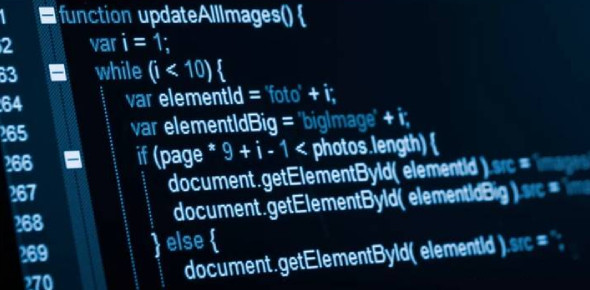# C Programming Basics: Quiz!

14 Questions | Attempts: 387Settings.

• 1.
What is the right way to initialize an array?​
• A.

Int num = { 2, 4, 12, 5, 45, 5 };

• B.

Int n{} = { 2, 4, 12, 5, 45, 5 };

• C.

Int n{6} = { 2, 4, 12 };

• D.

Int n(6) = { 2, 4, 12, 5, 45, 5 };

• 2.
What will be the output of the program?#include<stdio.h>void main(){    int a = {5, 1, 15, 20, 25};    int i, j, m;   i = ++a;   j = a++;   m = a[i++];   printf("%d, %d, %d", i, j, m);} ​
• A.

3, 2, 15

• B.

2, 3, 20

• C.

2, 1, 15

• D.

1, 2, 5

• 3.
What will be the output of following program code?#include <stdio.h>int main(void){    char p;   char buf = {1, 2, 3, 4, 5, 6, 9, 8};   p = (buf + 1);    printf("%d", p);   return 0;}
• A.

5

• B.

6

• C.

9

• D.

10

• 4.
An array elements are always stored in ________ memory locations.
• A.

Sequential

• B.

Random

• C.

Sequential and Random

• D.

None of the Above

• 5.
Let x be an array. Which of the following operations are illegal?I.    ++xII.    x+1III.    x++IV.    x*2
• A.

I and II

• B.

I, II and III

• C.

II and III

• D.

I, III and IV

• E.

III and IV

• 6.
Size of the array need not be specified, when-
• A.

Initialization is a part of definition

• B.

It is a declaration

• C.

It is a formal parameter

• D.

All of these

• 7.
Consider the following type definition.typedef char x;x myArray;What will sizeof(myArray) be ? (Assume one character occupies 1 byte)
• A.

15

• B.

10

• C.

50

• D.

30

• E.

None of the above

• 8.
What will be printed after execution of the following code?void main(){  int arr = {1,2,3,4,5};      printf("%d", arr);}
• A.

Garbage Value

• B.

5

• C.

6

• D.

0

• E.

None of the above

• 9.
What will be the output of the program if the array begins at 65472 and each integer occupies 2 bytes?#include<stdio.h>      void main(){    int a = {1, 2, 3, 4, 4, 3, 2, 1, 7, 8, 9, 0}; printf("%u, %u", a+1, &a+1);}
• A.

65474, 65488

• B.

65480, 65488

• C.

65480, 65496

• D.

65474, 65476

• 10.
What will happen after compiling and running following code?main(){     printf("%p", main);       }
• A.

Error

• B.

• C.

None of these

• D.

Will make an infinite loop

• 11.
Use of functions-
• A.

Enhances the logical clarity of the program.

• B.

Helps to avoid repeated programming across programs.

• C.

• D.

All of the above

• 12.
Find out the output:main(){     int i = abc(10);     printf("%d", --i);}int abc(int i){      return(i++);}
• A.

10

• B.

9

• C.

11

• D.

Compile time error

• 13.
What will be the output of the following program code?main(){     static int var = 5;     printf("%d ", var--);     if(var)           main();}
• A.

5 5 5 5 5

• B.

5 4 3 2 1

• C.

Infinite Loop

• D.

Compilation Error

• 14.
Which one do you like?
• A.

Option 1

• B.

Option 2

• C.

Option 3

• D.

Option 4

## Related TopicsBack to top
×

Wait!
Here's an interesting quiz for you.